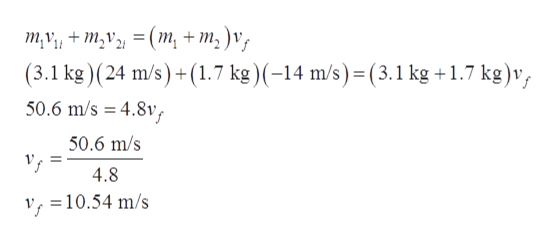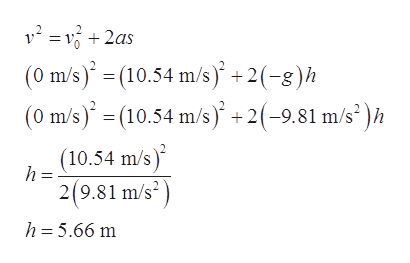# A completely inelastic collision occurs between two balls of wet putty that move directly toward each other along a vertical axis. Just before the collision, one ball, of mass 3.1 kg, is moving upward at 24 m/s and the other ball, of mass 1.7 kg, is moving downward at 14 m/s. How high do the combined two balls of putty rise above the collision point? (Neglect air drag.)

Question
91 views

A completely inelastic collision occurs between two balls of wet putty that move directly toward each other along a vertical axis. Just before the collision, one ball, of mass 3.1 kg, is moving upward at 24 m/s and the other ball, of mass 1.7 kg, is moving downward at 14 m/s. How high do the combined two balls of putty rise above the collision point? (Neglect air drag.)

check_circle

Step 1

From the conservation of momentum.help_outlineImage Transcriptioncloseту + m,V, 3 (т, + т,)v, (3.1 kg)(24 m/s)+(1.7 kg)(-14 m/s)-(3.1 kg +1.7 kg)v, = 4.8v, 50.6 m/s 50.6 m/s 4.8 v, =10.54 m/s fullscreen
Step 2

The combine mass moves toward upward. The motion of these balls are under gravity that is motion is under free fall. Therefore, acceleration is –g and the distance cov...help_outlineImage Transcriptionclose(0 m/s)(10.54 m/s) +2(-g)h (0 m/s)(10.54 m/s +2(-9.81 m/s2)h (10.54 m/s) h 2(9.81 m/s2 h 5.66 m fullscreen

### Want to see the full answer?

See Solution

#### Want to see this answer and more?

Solutions are written by subject experts who are available 24/7. Questions are typically answered within 1 hour.*

See Solution
*Response times may vary by subject and question.
Tagged in

### Collisions